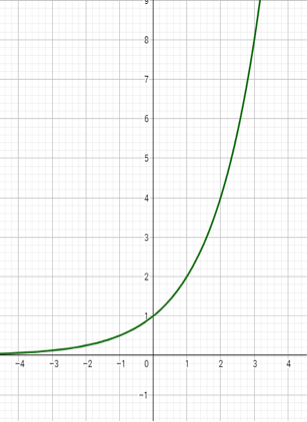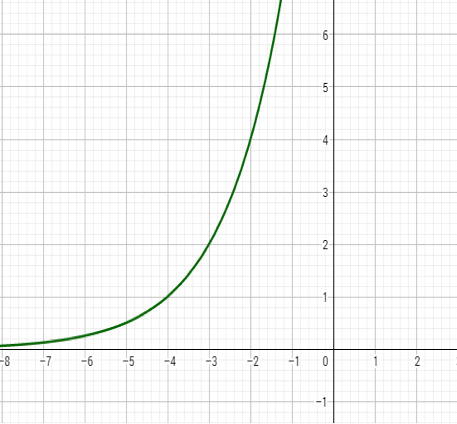# FNGR 1 | Lesson 4 | Explore (Horizontal Translations)## Horizontal Translations

A horizontal transformation occurs when we add or subtract a constant value to the inputs. It is represented as either $$f(x+h)$$ or $$f(x-h)$$.

Example:

The parent function for the exponential is $$f(x)=2^x$$:If we translate this graph left 4 units we end up with this graph:The new equation for the function is $$f(x)=2^{(x+4)}$$.

Examine the table of values for the original and the new functions:

$$x$$ $$f(x)=2^x$$
$$-2$$ $$\dfrac{1}{4}$$
$$-1$$ $$\dfrac{1}{2}$$
$$0$$ $$1$$
$$1$$ $$2$$
$$2$$ $$4$$
$$x$$j0 $$f(x)=2^{(x+4)}$$
$$-6$$ $$\dfrac{1}{4}$$
$$-5$$ $$\dfrac{1}{2}$$
$$-4$$ $$1$$
$$-3$$ $$2$$
$$-2$$ $$4$$

What do you notice? What do you wonder? Describe what you think is happening in your own words. How does this compare to the graphs above?

Graphs are created using Desmos.com.